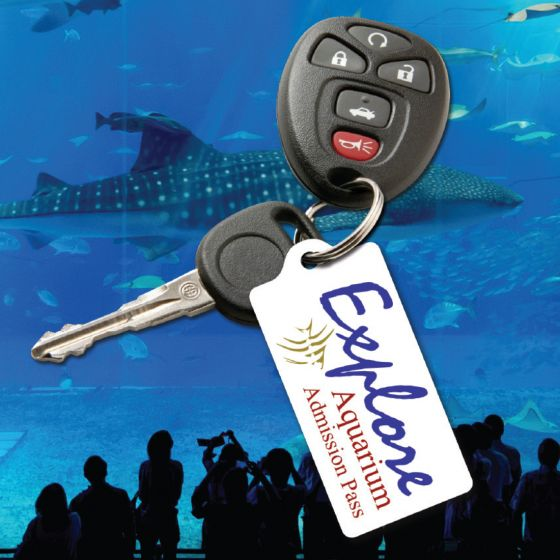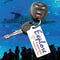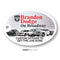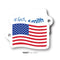Keep your dealership and service department phone number in customer's hand!

• Choose from a wide selection of shapes
• Includes 1" key rings
• Full color

Pricing:

One Side:

• 250= .74 each
• 500= .56 each
• 1000= .41 each
• 2500= .29 each
• 5000= .19 each

Two Sides:

• 250= .78 each
• 500= .59 each
• 1000= .45 each
• 2500= .33 each
• 5000= .22 each

*\$25 Setup Charge

*3-4 week production time

*All orders subject to +/- 5% overrun